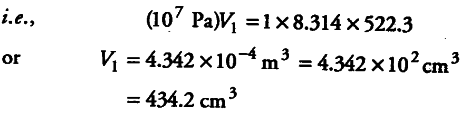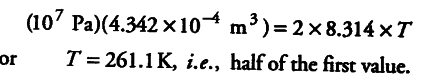# 1 mole of an ideal gas undergoes reversible isothermal expansion

1 mole of an ideal gas undergoes reversible isothermal expansion from an initial volume of \${ V }{ 1 }\$ to a final volume of 10
\${ V }
{ 1 }\$ and does 10 kJ of work. The initial pressure was 1 x \${{10}^{7}}\$ Pa.
(i) Calculate \${ V }_{ 2 }\$
(ii) If there were 2 moles of gas, what must its temperature have been?

(i) We know that W = — 2.303 nRT logV2/V1
10 x {{10}^{3}} J = 2.303 x 1 x 8.314 x T x log 10V1/V1
T = 522.3K
For initial conditions, p1V1 = n1RTIf there were 2 moles of the gas, applying p1 V1 = n1 RT, we get